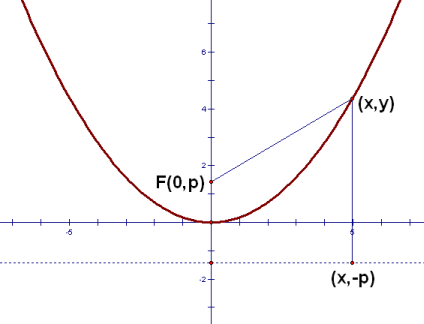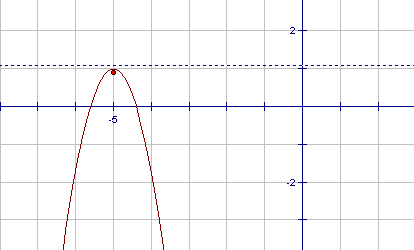﻿ Equations of Simple Parabolas
EQUATIONS OF SIMPLE PARABOLAS
• PRACTICE (online exercises and printable worksheets)
• You may want to review the prior web exercise, Parabolas.

If a parabola is placed in a coordinate plane in a simple way, then a simple equation is obtained.

## Derivation

 Place a parabola with its vertex at the origin, as shown at right. If we put the focus on the $\,y$-axis, then the directrix will be parallel to the $\,x$-axis. Or, if we put the directrix parallel to the $\,x$-axis, then the focus will be on the $\,y$-axis. In either case, let $\,p \ne 0\,$ denote the $\,y$-value of the focus. Thus, the focus has coordinates $\,(0,p)\,$. Although the sketch at right shows the situation where $\,p\gt 0\,$, the following derivation also holds for $\,p \lt 0\,$.Notice that:

 $p\gt 0$ if and only if the focus is above the vertex if and only if the parabola is concave up (holds water) AND $p\lt 0$ if and only if the focus is below the vertex if and only if the parabola is concave down (sheds water)

For example, if the focus is above the vertex, then $\,p\,$ must be greater than zero, and the parabola must be concave up.
As a second example, if the parabola is concave down, then $\,p\,$ must be less than zero, and the focus is below the vertex.

Since the vertex is a point on the parabola, the definition of parabola dictates that it must be the same distance from the focus and the directrix.

Thus, the directrix must cross the $\,y\,$-axis at $\,-p\,$; indeed, every $\,y$-value on the directrix equals $\,-p\,$.

Let $\,(x,y)\,$ denote a typical point on the parabola.

The distance from $\,(x,y)\,$ to the focus $\,(0,p)\,$ is found using the distance formula: $$\tag{1} \sqrt{(x-0)^2 + (y-p)^2 } = \sqrt{x^2 + (y-p)^2}$$

To find the distance from $\,(x,y)\,$ to the directrix, first drop a perpendicular from $\,(x,y)\,$ to the directrix.
This perpendicular intersects the directrix at $\,(x,-p)\,$.
The distance from $\,(x,y)\,$ to the directrix is therefore the distance from $\,(x,y)\,$ to $\,(x,-p)\,$: $$\tag{2} \sqrt{(x-x)^2 + ((y-(-p))^2} = \sqrt{(y+p)^2}$$

From the definition of parabola, distances $\,(1)\,$ and $\,(2)\,$ must be equal: $$\sqrt{x^2 + (y-p)^2} = \sqrt{(y+p)^2}$$

This equation simplifies considerably, as follows:

 Squaring both sides: $x^2 + (y-p)^2 = (y+p)^2$ Multiplying out: $x^2 + y^2 - 2py + p^2 = y^2 + 2py + p^2$ Subtracting $\,y^2 + p^2\,$ from both sides: $x^2 - 2py = 2py$ Adding $\,2py\,$ to both sides: $x^2 = 4py$ Dividing by $\,4p\,$ and rearranging: $\displaystyle y = \frac{1}{4p} x^2$

Such a beautiful, simple description for our parabola!
The most critical thing to notice is the coefficient of $\,x^2\,$, since it holds the key to locating the focus of the parabola.

As an example, consider the equation $\,y = 5x^2\,$.
Comparing $\,y = 5x^2\,$ with $\displaystyle \,y = \frac1{4p}x^2\,$, we see that $\displaystyle 5 = \frac{1}{4p}\,$.
Solving for $\,p\,$ gives:

\begin{alignat}{2} 5\ &= \frac{1}{4p} &\qquad&\text{original equation} \cr 20p\ &= 1 &&\text{multiply both sides by } 4p\cr p\ &= \frac{1}{20} &&\text{divide both sides by } 20 \end{alignat}

Thus, $\,y = 5x^2\,$ graphs as a parabola with vertex at the origin, and focus $\displaystyle\,(0,\frac{1}{20})\,$.
So easy!

In summary, we have:

EQUATIONS OF SIMPLE PARABOLAS

Every equation of the form $\,y= ax^2\,$ (for $\,a\ne0\,$) is a parabola
with vertex at the origin, directrix parallel to the $\,x\,$-axis, and focus on the $\,y\,$-axis.

If $\,a\gt 0\,$, then the parabola is concave up (holds water).
If $\,a\lt 0\,$, the the parabola is concave down (sheds water).

If $\,p\,$ denotes the $\,y$-value of the focus, then $\displaystyle\,a = \frac{1}{4p}\,$.
Solving for $\,p\,$ gives $\,\displaystyle p = \frac{1}{4a}\,$, and thus the coordinates of the focus are $\displaystyle \,(0,\frac{1}{4a})\,$.

MEMORY DEVICE: ‘one over four pee pairs’

Notice that if $\displaystyle\,a = \frac{1}{4p}\,$, then $\displaystyle\,p = \frac{1}{4a}\,$.   Or, if $\displaystyle\,p = \frac{1}{4a}\,$, then $\displaystyle\,a = \frac{1}{4p}\,$.   What a beautiful symmetric relationship!

Thus, I fondly refer to $\,a\,$ and $\,p\,$ with the catchy phrase:   ‘one over four pee pairs’. (Try to say this quickly ten times in a row!)
If you know either $\,a\,$ or $\,p\,$, then it's easy to find the other—just multiply by $\,4\,$ and then flip (take the reciprocal).

For example, if you know that $\,a = 5\,$, then $\,p\,$ is found as follows:

• $\,4\times 5 = 20\,$       (multiply by $\,4\,$)
• $\displaystyle p=\frac{1}{20}$       (flip)
Or, if you know that $\displaystyle\,p = \frac{1}{20}\,$, then $\,a\,$ is found as follows:
• $\displaystyle\,4\times \frac{1}{20} = \frac{4}{20} = \frac{1}{5}\,$       (multiply by $\,4\,$)
• $\displaystyle a = (\text{the reciprocal of } \frac{1}{5}) = 5\,$       (flip)

SHIFTING THE PARABOLA

Now, here's some very good news.
By using graphical transformations, knowledge of this one simple equation $\,y = ax^2\,$
actually gives full understanding of all parabolas with directrix parallel to the $\,x$-axis!
The results are summarized next:

Shift the parabola (together with its focus and directrix) horizontally by $\,h\,$, and vertically by $\,k\,$.
This yields the following information:
 original equation: $y=ax^2$ shifted equation: $y=a(x-h)^2 + k$ original vertex: $(0,0)$ new vertex: $(h,k)$ original focus: $\displaystyle (0,p) = (0,\frac{1}{4a})$ new focus: $\displaystyle (h,p+k)= (h,\frac{1}{4a}+k)$ original directrix: $y=-p$ new directrix: $y=-p+k$

EXAMPLE graphing a shifted parabola

In this example, I illustrate the approach that I usually take when asked to give
complete information about the parabola $\,y = a(x-h)^2 + k\,$.

Question:
Completely describe the graph of the equation $\,y = -3(x+5)^2 + 1\,$.
Solution:
• Concave up or down?
Since $\,a=-3\lt 0\,$, the parabola is concave down (sheds water).
Since the focus is always inside a parabola, we know the focus is under the vertex.
• Find the vertex:
The vertex of   $\,y = a(x-h)^2 + k\,$   is the point $\,(h,k)\,$:
$$y = a( \overset{\text{What value of } \ x\ }{\overset{\text{ makes this zero?}}{\overbrace{ \underset{\text{Answer: } \ h}{\underbrace{x-h}}}}})^2\ \ \ + \overset{\text{this is the } y\text{-value of the vertex}}{\overbrace{\ \ k\ \ }}$$ For us, what makes $\,x+5\,$ equal to zero? Answer: $\,-5\,$
Thus, $\,-5\,$ is the $\,x$-value of the vertex.
When $\,x = -5\,$, the corresponding $\,y$-value is $\,1\,$.
Thus, the vertex is $\,(-5,1)\,$.
• Find the distance from the vertex to the focus:
Using the ‘one over four pee pair’ memory device,
$\displaystyle\,p = \frac{1}{4a} = \frac{1}{4(-3)} = -\frac{1}{12}\,$.
Thus, the distance from the focus to the vertex is $\displaystyle\,|p| = \left|-\frac1{12}\right| = \frac{1}{12}\,$.
• Find the focus:
We already determined that the focus lies below the vertex.
So, the focus is:
$\displaystyle\,(-5,1-\frac{1}{12}) =(-5,\frac{11}{12})\,$
• Find the equation of the directrix:
Every horizontal line has an equation of the form:   $\,y = \text{(some #)}\,$
In our example, the focus lies $\displaystyle\,\frac{1}{12}\,$ below the vertex, so the directrix lies $\displaystyle\,\frac{1}{12}\,$ above the vertex.
Thus, the equation of the directrix is $\displaystyle\,y=1+\frac{1}{12}\,$, that is, $\displaystyle\,y=\frac{13}{12}\,$.
Plotting an additional point gives a sense of the ‘width’ of the parabola.
For example, when $\,x = -4\,$ we have:
$\,y = -3(-4+5)^2 + 1 = -3(1) + 1 = -2\,$
In this parabola, the vertex is close to the focus, so the parabola is narrow.$\,y=-3(x+5)^2+1\,$

For fun, zip up to WolframAlpha and type in any of the following:

vertex of y = -3(x+5)^2 + 1

focus of y = -3(x+5)^2 + 1

directrix of y = -3(x+5)^2 + 1

How easy is that?!

Master the ideas from this section